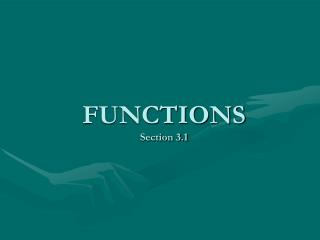DownloadDownload PresentationFUNCTIONS Section 3.1

# FUNCTIONS Section 3.1

Download Presentation## FUNCTIONS Section 3.1

- - - - - - - - - - - - - - - - - - - - - - - - - - - E N D - - - - - - - - - - - - - - - - - - - - - - - - - - -
##### Presentation Transcript

1. FUNCTIONSSection 3.1

2. RELATIONS • Definition: A relation is a correspondence between two sets. • If x and y are two elements in these sets and if a relation exists between x→y, then we say that “x” correspond to “y” or that “y” depends on “x”

3. Example: Y= 3X – 1 If X =2 then Y= 3.2 – 1 So, y=5

4. A map: illustrate a relation by using a set of inputs and drawing arrows to the corresponding elements in the set of outputs. Ordered pairs can be used to represent x→y as ( x , y ) { (0,-2),(0,1),(1,2),(2,1), (3,4)}

5. Determine whether a relation represents a Function Let X and Y be two nonempty sets. “ A function from X into Y is a relation that associates with each element of X exactly one element of Y”

6. Let’s now use ordered pairs to identify which of these sets are relations or functions: • {(1,4) , (2,5) , (3,6), (4,7)} Do={1,2,3,4} Rg={4,5,6,7} • {(1,4),(2,4)(3,5),(6,10)} Do={1,2,3,6} Rg={4,5,10} • { (-3,9), (-2,4), (0,0), (1,1), (-3,8)}

7. Determine whether an Equation is a Function Determine if the equation y=2x – 5 defines y as a function of x If x=1, then y=2(1) – 5 = -3 If x=3, then y= 2(3) – 5 = 1 The equation is a FUNCTION

8. Example 2 Determine if the equation x2+y2=1 defines y as a function of x. Solve for y: y2= 1 - x2 y= ± \̸ 1-x2 If x=0 then y = ±1 This means the equation x2+y2=1 does not define a function

9. Find the value of a Function y = f(x) read “f of x” Example: y=f(x) = 2x – 5 then f(1/2)=2.1/2 – 5 f(1/2)= -4 The variable x is called independent variable or argument, and y is called dependent variable

10. Finding the Domain of a Function The domain of a function is the largest set of Real numbers for which the value f(x) is a Real number. Examples: Find the domain of each of the following functions: (a) f(x)= x2+5x (b) g(x)= 3x . x2-4 (c) h(t) = \̸ 4-3t

11. Solutions: • (a) Domain of f is the set of all Real Numbers. • (b) Domain of g is {x ̸ x ≠±2} • (c) Domain of h is { t ̸ t≤4/3}

12. Tips to find the Domain of a function • Start with the domain as the set of real numbers. • If the equation has a denominator, exclude any numbers that give a zero denominator. • If the equation has a radical of even index, exclude any numbers that cause the expression inside the radical to be negative.

13. SUMMARY • Function: a relation between two sets of real numbers so that each number x in the first set, the domain, has corresponding to it exactly one number y in the second set, the range. • Unspecified Domain: If a function f is defined by an equation and no domain is specified, then the domain will be taken to be the largest set of real numbers for which the equation defines a real number. • Function Notation: y= f(x) f is the symbol for the variable, x is the independent variable or argument, y is the dependent variable, and f(x) is the value of the function at x, or the image of x.

14. GAME TIME 10 10 20 20 30 30 40 40

15. Do = { 1, 3, 4}

16. f(x) = 2X + 1 ANSWER

17. Do= all real numbers

18. g(x) = 1 . X - 1 ANSWER

19. Do ={ X/ X≠1}

20. . . • h(x) = √ X-2 ANSWER

21. Do={ x/x ≥ 2}

22. Rg = { 2, 4, 0}

23. f(x) = 2X + 1 ANSWER

24. Rg = all real numbers

25. g(x) = 1 . X - 1 ANSWER

26. Rg = {x/x≠0}

27. . . • h(x) = √ X-2 ANSWER

28. Rg={x/x≥0}